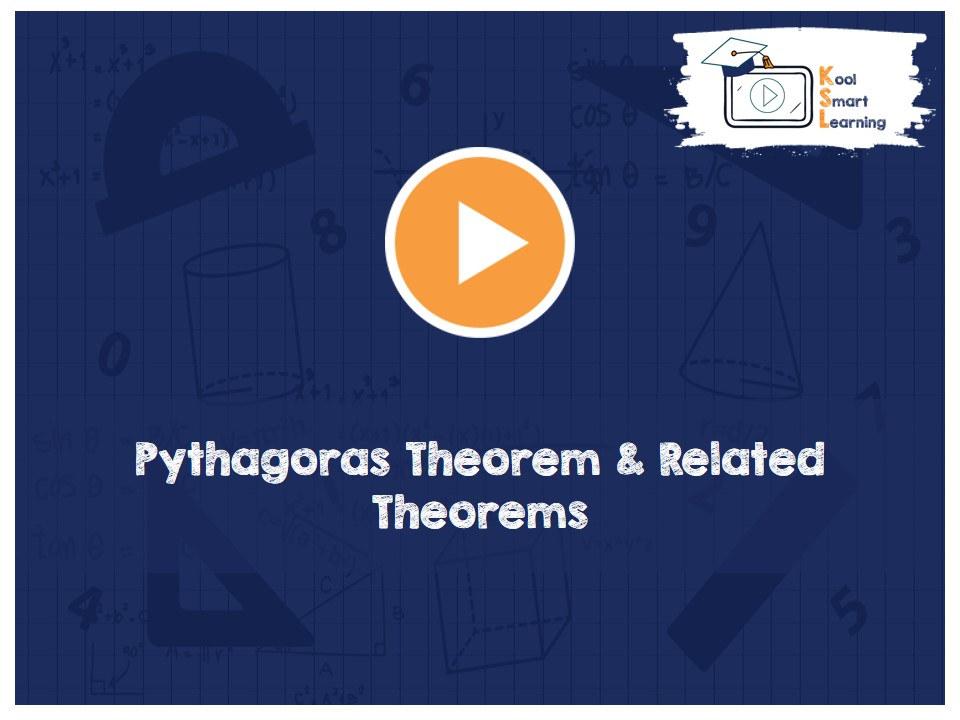At KoolSmartLearning, we intend to harness the power of online education to make learning easy.## Pythagoras Theorem & Related Theorems

/  Pythagoras Theorem & Related Theorems## Pythagoras Theorem & Related Theorems

The video discusses Pythagoras Theorem & its converse theorem. The video also includes other related Theorem for acute angle triangles and obtuse angle triangles along with their proof. The theorem for the relation between any two sides of a triangle and the third side together with median is also covered along with its detailed proof.

More Related Videos